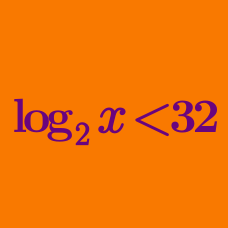Algebra

# Logarithmic Inequalities: Level 2 Challenges

It is well known that $$\ln 2 < \ln 3$$. Which of the following is bigger:

$[ \ln ( \ln 2 ) ] ^2 \text { or } [ \ln ( \ln 3) ]^2 ?$

Find the range of positive value of $$x$$ such that $$\log_3 (x+7) < \log_9(x^2+77)$$ is fulfilled?

Which of the following logarithms is greater?$\color {green}{\log _{9}{71}} ~~~~\text{or}~~~~\color {blue}{\log _{8}{61}}$

$\ln\left(2x^{2} - 3x + 8\right) \le \dfrac{\ln\left(x^{4}+4x^{3} + 8x^{2} + 8x + 4\right)}{2}$

Find the product of all integer values of $$x$$ which satisfy the inequality above.

True or false:

For $$1<x<2$$, the inequality $$\log_{10} (x+99) > x$$ is satisfied.

×